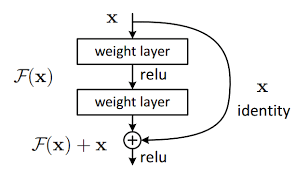Hello,
I am trying to do set-matching. meaning given set_a and set_b a model M can generate a similarity score between these sets. M(set_a, set_b) = alpha and alpha is positive float. I wanted to start with this baseline. The gradients are all in magnitude of 1.e-6 so there’s no learning, and the code is pretty ugly (for loops) and I think that might be the reason behind this gradients.

``````class MyMatcher(nn.Module):
def __init__(self, node_features):
super(MyMatcher, self).__init__()
self.device = torch.device(
'cuda' if torch.cuda.is_available() else 'cpu')
self.node_encoder = nn.Sequential(nn.Linear(node_features, 128), nn.ReLU(), nn.Linear(128, 128), nn.ReLU(), nn.Linear(128, 128), nn.ReLU())
self.self_attention = nn.Sequential(nn.Linear(128*2, 128), nn.Tanh(), nn.Linear(128, 1))

def cosine_sim(self, a, b):
a_norm = a / a.norm(dim=1)[:, None]
b_norm = b / b.norm(dim=1)[:, None]
def similarity(self, set_a, set_b):
sim = self.cosine_sim(set_a, set_b)
rows, columns = linear_sum_assignment(1 - sim.cpu().detach().numpy())
selected_b = set_b[columns]
selected_a = set_a[rows]
input_attention = torch.hstack([self.node_encoder(selected_a), self.node_encoder(selected_b)])
output_attention = F.softmax(self.self_attention(input_attention), dim=0)
def forward(self, x, y, num_candidates):
scores = torch.empty((x.shape,num_candidates - 1), dtype=torch.float32).to(self.device)
for batch in range(len(x)):
scores[batch] = self.forward_single_batch(x[batch:batch+1], y[batch:batch+1])
return scores
def forward_single_batch(self, x, y):
assert x.shape == 1
x, y = x[y!=-1], y[y!=-1] # remove batch indice, remove padded data
x_anchor = x[y==0]
anchor_len = len(x_anchor)
scores = torch.empty((1,len(torch.unique(y[y!=0]))), dtype=torch.float32).to(self.device)
sorted_y, _ = torch.sort(torch.unique(y[y!=0]))
for idx, yn in enumerate(sorted_y):
temp_xn  = x[y==yn]
if len(temp_xn) < anchor_len:
scores[0, idx] = self.similarity(set_a =temp_xn , set_b = x_anchor)
else:
scores[0, idx] = self.similarity(set_a = x_anchor, set_b = temp_xn)
return scores
``````

The inputs (x, y, num_candidates):

x: is the shape of (batch_size, N, set_element_feature_size). a single batch contains 7 sets, and every set might have a different number of elements in it. so for example, batch1 might have 230 elements, batch2 118 elements… to be able to collate the batches, I bad with 0 smaller batches. and end up in this case with (2, 230, set_element_feature_size).

y: is the shape (batch_size, N) is an indexer for x. every value of y tells us to which set a given row in x belongs. so since we have 7 sets the values of y are in {0, 1, 2, 3, 4, 5, 6, -1} the -1 here represents the row that we padded in x during the collate function.

My question is:

• from a gradient point of view and not from “pretty not pretty code” point of view. Do you see any problem that might cause gradients to be small.
• Do you see a way to vectorise this ?
• The gradients are not good in the last layer and then they vanish when we go deeper. the gradients are all in the magnitude of 1e-6, 1.e-8, 1.e-9 and some are 0.

Thank you

Hello ilyes!

…could you try the ResNet approach for your `node_encoder` below?

It would require replacing your sequence of linear and relus with blocks like the below, in diagram:The idea is that if the natural starting point for your transformations is the identity, then learning just the difference from the identity (rather than having to learn to converge towards the identity from a random starting point) is much less likely to lead to vanishing gradients, and generally allows for deeper networks (in ConvNets at least). You can read more from a quick Google.

I don’t undertand your problem well enough to know whether this solution is applicable, but if you weren’t aware of it I think it’s worth giving it a think, given how much it helps in ConvNets.

Good luck!
Andrei

EDIT: Relatedly, you may consider adding batch normalization or something equivalent to it, to the extent it’s possible for your problem, as this typically also helps with avoiding vanishing gradients in other scenarios.